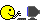# Merge maps with sort

I have two lists. one is Lang list with id. Another is sort order list with id.

``````    lang_list = [%{id: 1, name: "Elixir"}, %{id: 2, name: "Java"}, %{id: 3, name: "Ruby"}, %{id: 4, name: "Python"}]
sort_list = [%{id: 3, sort: 1}, %{id: 2, sort: 2}, %{id: 4, sort: 3}, %{id: 5, sort: 4}, %{id: 1, sort: 5}]
``````

I would like to merge both lists like following result.

``````result_list = [%{id: 3, name: "Ruby", sort: 1},
%{id: 2, name: "Java", sort: 2},
%{id: 4, name: "Python", sort: 3},
%{id: 1, name: "Elixir", sort: 5}
]
``````

What can I do for it?

1. Merge sort id in last with same id.
2. Sort maps by sort id

I’d just append, group, merge, sort…

Roughly like this:

``````Enum.group_by(lang ++ sort, &(&1.id))
|> Enum.map(fn {_, [a, b]} -> Map.merge(a, b) end)
|> Enum.sort_by(&(&1.sort))
``````
1 Like

It might be possible to use an even more optimized algorithm, but for that we need some more information:

• How large are these lists in reality? Will they get longer and longer or are they of a fixed size?
• Is the `sort` field always filled with consecutive numbers, or might it contain gaps?
1 Like

What if it has extra id in sort_list like this sample %{id: 5, sort:4} which contains id gaps?
I would like to avoid this error.

`````` The following arguments were given to anonymous fn/1 in XXXX/0:
# 1
{5, [%{id: 5, sort: 4}]}
``````
• How large are these lists in reality? Will they get longer and longer or are they of a fixed size?

These lists are more than thousands. not fixed size.

• Is the `sort` field always filled with consecutive numbers, or might it contain gaps?

Yes I have to consider contain gaps.

``````  def merge_and_sort_language_pairs(lang_list, sort_list) do
lang_list ++ sort_list
|> Enum.group_by(& &1.id)
|> Enum.filter(fn({_k, v}) -> length(v) > 1 end)
|> Enum.map(fn({_id, lang_fragments}) ->
Enum.reduce(lang_fragments, fn(lang, acc) ->
Map.merge(acc, lang)
end)
end)
|> Enum.sort_by(& &1.sort)
end
``````

Homework: decipher it.Hmm. In that case, doing clever Radix-based solutions are out.

In this case I would already say that appending these two lists would be a bad idea, since this takes linear time based on the size of the first list.

My shot:

``````defmodule Foo do

@doc """
Combines two lists whose values are both supposed to be maps or structs that have an `.id`-field.
Merges each of these maps/structs when they have the same `.id`,
and returns the result as a list ordered by the `.sort`-field
that these maps/structs are also expected to have.
"""
def combine_lists_of_maps_with_same_ids(list_a, list_b) do
Map.merge(list_to_id_map(list_a), list_to_id_map(list_b), fn _, map1, map2 ->
Map.merge(map1, map2)
end)
|> Map.values
|> Enum.sort_by(&(&1.sort))
end

@doc """
Turns a list whose fields are maps or structs that have an `.id`-field,
into a map where the keys are the values of these `.id`-fields.
"""
def list_to_id_map(input) do
for x = %{id: id} <- input, into: %{}, do: {id, x}
end
end
``````
``````def list_to_id_map(input) do
for x = %{id: id} <- input, into: %{}, do: {id, x}
end
``````

One thing I don’t like about `for` comprehensions is how I read them:

1. `for x = %{id: id} <- input` from the beginning but read right to left
2. `do: {id, x}` at the end
3. `into: %{}` in the the middle

Makes me fell positively dyslexic; it’s enough to make me instead go with:

``````def to_id_kv(%{id: id} = x),
do: {id, x}

def list_to_id_map(list),
do: map.new(list, &to_id_kv/1)
``````

Your version leaves in records that have `id` and `sort` but do not have `name`, and I was left with the impression that the OP does not want that. (My code only leaves those records that have all 3 fields after a merge.)

Thank you all !!
My understanding is very slow. However I will try to catch up with your all advice step by step. It’s so helpful of you.